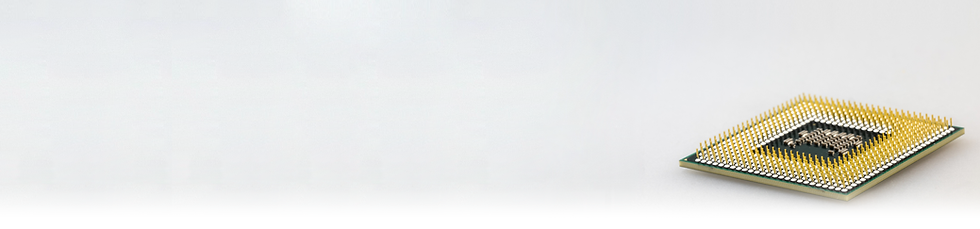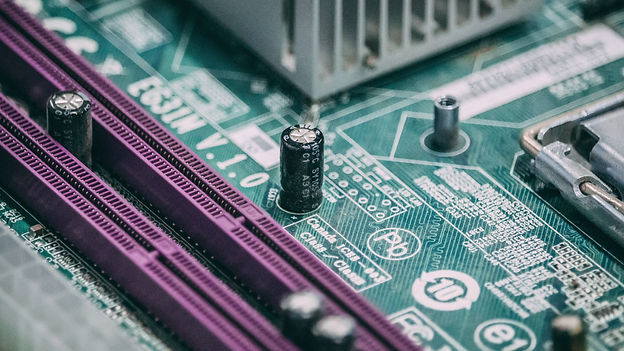top of page# What is Si-Cap ?A capacitor is a device that stores electrical energy in an electric field. It consists of two conductive plates separated by an insulating material called a dielectric. When a voltage is applied across the plates, the capacitor charges up and stores electrical energy. The amount of energy stored in the capacitor is directly proportional to the voltage applied and the capacitance of the capacitor.

## Conventional CapacitorA conventional capacitor is a type of capacitor that uses a dielectric material to separate two conductive plates. The dielectric material can be made of materials such as ceramic, paper, or mica, and it determines the capacitance value of the capacitor. The two conductive plates are usually made of metal, saluminum or copper, and are separated by the dielectric material. When a voltage is applied to the capacitor, electrical energy is stored in the electric field between the two plates.

## Common types of Conventional capacitors

Conventional capacitors are widely used in electronic circuits for a variety of applications, such as filtering, timing, and energy storage. They are available in a wide range of capacitance values, voltage ratings, and sizes, making them suitable for use in many different types of circuits.Electrolytic capacitors

##### these capacitors use a liquid electrolyte as the dielectric and are polarized, meaning that they must be connected with the correct polarity to function properly. They are commonly used in power supply circuits.Film capacitors

##### these capacitors use a thin film of metal or metal oxide as the dielectric and are non-polarized, meaning that they can be connected in either direction. They are commonly used in high-frequency circuits.Ceramic capacitors

these capacitors use a ceramic material as the dielectric and are available in both polarized and non-polarized versions. They are widely used in electronic circuits due to their low cost and stability over time. Currently, Multilayer Ceramic Capacitor (MLCC) is the most widely used ceramic capacitor type.

## Silicon Capacitor (Si-Cap)A silicon capacitor is a type of capacitor that uses a silicon semiconductor material as its main substrate. It typically uses high-k dielectric allowing a high capacitance per unit area, making silicon capacitors ideal for use in integrated circuits where space is limited. The capacitance of a silicon capacitor is dependent on the area of the dielectric and the thickness of the oxide layer.

Low temperature coefficient of capacitance their capacitance does not change much with changes in temperature.

Low leakage current, Silicon capacitor can store electrical energy for long periods without significant loss.

Ideal for use in applications that require high precision and stability over time.

## Usage

High-precision applications such as frequency control, voltage reference, timing circuits.

Oscillators, filters, and other circuits where stable capacitance values are required.

RF (radio frequency) circuits, as they have low parasitic effects such as inductance and resistance that can affect the performance of the circuit.

Integrated circuits,  discrete components in electronic circuits.

bottom of page